# Demo: Alternating Projections

Our goal is to find a point in the intersection of two convex sets $$C_1$$ and $$C_2$$. A similar goal is to find the projection of an arbitrary point onto the intersection; or, if the intersection is empty, find the point(s) in $$C_1$$ that are as close as possible to $$C_2$$. In this demo, the intersection of $$C_1$$ and $$C_2$$ is a singleton, so the concepts are the same.

We assume that we know how to project on the sets $$C_1$$ and $$C_2$$ individually, but not onto $$C_1\cap C_2$$.

The basic algorithm to solve this problem is the alternating projection method, first studied by John von Neumann for the case where $$C_1$$ and $$C_2$$ are affine spaces. This algorithm can be extended to arbitrary convex sets, although you may not converge to the projection of the original point. The algorithm is very simple: starting at some point $$y$$, and with $$x\leftarrow y$$, we update $$x \leftarrow \text{Proj}_{C1}(\text{Proj}_{C2}(x))$$, where $$\text{Proj}_{C1}$$, $$\text{Proj}_{C2}$$ are the projectors onto the sets $$C_1$$ and $$C_2$$, respectively.

Better methods (which actually give you the projection of y onto the intersection) are based on Dykstra’s algorithm (not to be confused with the Dijkstra algorithm described here on Wikipedia). Dykstra’s algorithm also uses only $$\text{Proj}_{C1}$$ and $$\text{Proj}_{C2}$$, but with a few intermediate steps. For an overview, see the book Alternating Projection Methods by Escalante and Raydan.

Another overview of both alternating projection and Dykstra are in the book chapter Proximal splitting methods in signal processing (PDF) by P. L. Combettes and J.-C. Pesquet, in the book ‘Fixed-Point Algorithms for Inverse Problems in Science and Engineering’, (ed.: H. H. Bauschke, R. S. Burachik, P. L. Combettes, V. Elser, D. R. Luke, and H. Wolkowicz, Editors), pp. 185-212. Springer, New York, 2011.

The purpose of this demo is to show the above two methods, and also how to formulate this with TFOCS. The advantage of solving this with TFOCS is that we can use an accelerated solver (Nesterov-style) and use large step-sizes and line search, whereas Dykstra has no step-size parameter. In the first example, it is especially apparent that TFOCS avoids the slow convergence that both the alternating projection and Dykstra algorithms suffer from. In the second example, the performance of alternating projections and TFOCS are quite similar.

## Mathematical formulation

For a given point y, and two convex sets C1 and C2, we wish to find the closest point x to y, such that x is in both C1 and C2. We write this as:

$\textrm{minimize}_{x\in C_1 \cap C_2} \,\, \mu/2||x\textrm{–}y||_2^2$

The parameter $$\mu>0$$ is arbitrary and does not affect the answer.

This fits naturally into the TFOCS formulation since the primal problem is already strongly convex.

## Demo with two polygon sets in 2D that intersect at (0,0)

Set the dimension to 2

N = 2;  % 2D is best for visualization

Define some 2D polygons

mx = 1;
x1a = [1;.1];
x1b = [1;.2];
xx1 = [0,x1a(1),2*mx,x1b(1)];
yy1 = [0,x1a(2),2*mx,x1b(2)];

x2a = [1;.3];
x2b = [1;.35];
xx2 = [0,x2a(1),2*mx,x2b(1)];
yy2 = [0,x2a(2),2*mx,x2b(2)];

The solution is at x = (0,0), so our error function is simple

err = @(x) norm(x);

plot the regions

figure
red = [255,153,153]/255;
blue = [204,204,255]/255;
fill( xx1, yy1,red);
hold on
fill( xx2, yy2,blue);

xlim( [0,mx] ); ylim( [0,.5*mx] );
axis equal
text(.5,.07,'set C1');
text(.5,.22,'set C2');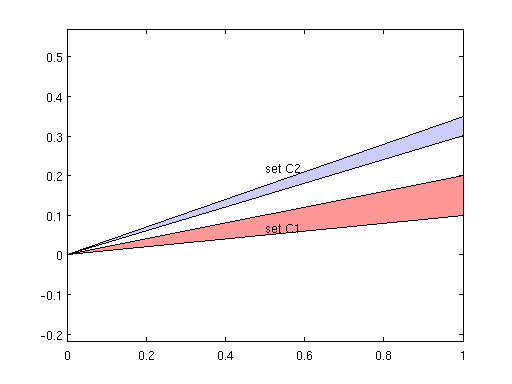Make some operators that will be used with TFOCS

addpath ~/Dropbox/TFOCS/  % modify this to wherever it is installed on your computer
op1     = project2DCone(x1a, x1b );
op2     = project2DCone(x2a, x2b );

offset1 = 0;
offset2 = 0;
op1_d   = prox_dualize(op1);
op2_d   = prox_dualize(op2);

% and some simpler operators that we will use with alternating projections
% and with Dykstra
proj1   = @(x) callandmap( op1, 2, x, 1); % gamma is irrelevant
proj2   = @(x) callandmap( op2, 2, x, 1 ); % gamma is irrelevant

% for all methods, we need to specify a starting point

x0      = [1; 1/4];

## solve in TFOCS

We can pick any mu and should get the same result, although due to some scaling issues in stopping criteria, it does have a small effect.

mu = 1e-6;

opts    = struct('debug',false,'printEvery',20,'maxIts',200);
opts.errFcn{1}  = @(f,d,x) err(x);
opts.errFcn{2}  = @(f,d,x) recordPoints(x);
recordPoints(); % zero-out counter. We use this to plot the points later

opts.tol        = 1e-15;

affineOperator  = {eye(N),offset1;eye(N),offset2};
dualProx        = {op1_d,op2_d};
% Solve in TFOCS:
[x,out,optsOut] = tfocs_SCD( [], affineOperator, dualProx , mu, x0, [], opts );

% Record the path:
path    = recordPoints();
path    = [x0,path];

figure
subplot(1,2,1);
fill( xx1, yy1,red);
hold on
fill( xx2, yy2,blue);
xlim( [0,mx] ); ylim( [0,.5*mx] );
text(.5,.07,'set C1');
text(.5,.22,'set C2');
plot( path(1,:), path(2,:),'ko-','linewidth',2 )
title('Path for TFOCS solving the intersection of 2 polygons');

subplot(1,2,2);
semilogy( out.err(:,1) ,'o-'); xlabel('iterations'); ylabel('error');
title('Error for TFOCS method');
set(gcf, 'Position', [400 200 800 400]);
Auslender & Teboulle's single-projection method
Iter    Objective   |dx|/|x|    step      errors
----+----------------------------------+-------------------
20  |         +Inf  2.90e-13  1.91e-07*| 5.64e-07 0.00e+00
31  |         +Inf  1.33e-16  1.39e-07*| 4.56e-08 0.00e+00
Finished: Step size tolerance reached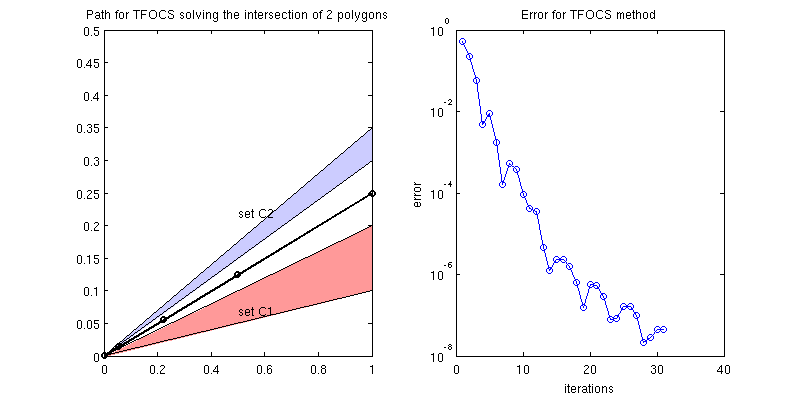## solve via alternating projection method

maxIts  = 500;
x       = x0;
path    = [x0];
errHist = [];
for k = 1:maxIts
% basic alternating projection method:
x   = proj1(x);
path= [path, x];
x   = proj2(x);
path= [path, x];
errHist     = [ errHist; err(x) ];
if ~mod(k,50)
fprintf('Iter %4d, error is %.2e\n', k, errHist(end) );
end
end
figure
subplot(1,2,1);
fill( xx1, yy1,red);
hold on
fill( xx2, yy2,blue);
xlim( [0,mx] ); ylim( [0,.5*mx] );
text(.5,.07,'set C1');
text(.5,.22,'set C2');
plot( path(1,:), path(2,:),'ko-','linewidth',1 )
title('Path for alternating projection method solving the intersection of 2 polygons');

subplot(1,2,2);
semilogy( errHist, 'o-' ); xlabel('iterations'); ylabel('error');
errHist_1 = errHist;
title('Error for alternating projection method');
set(gcf, 'Position', [400 200 800 400]);
Iter   50, error is 6.64e-01
Iter  100, error is 4.26e-01
Iter  150, error is 2.74e-01
Iter  200, error is 1.76e-01
Iter  250, error is 1.13e-01
Iter  300, error is 7.25e-02
Iter  350, error is 4.65e-02
Iter  400, error is 2.99e-02
Iter  450, error is 1.92e-02
Iter  500, error is 1.23e-02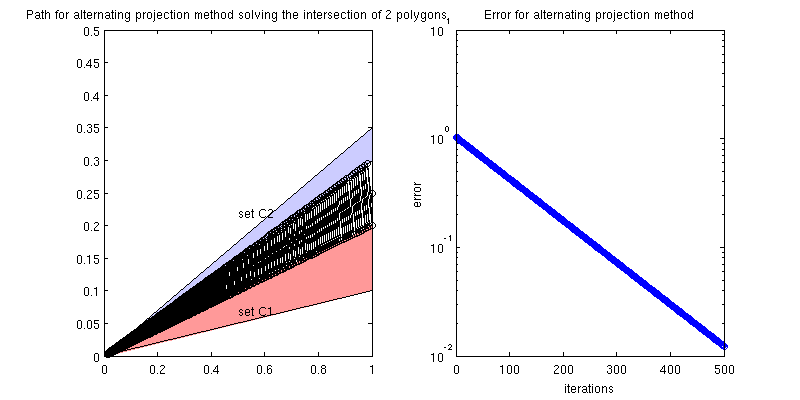With alternating projections, we get very slow convergence because of the very low angle between the two sets. Here is a zoom in on the graph of the iterates:

figure
fill( xx1, yy1,red);
hold on
fill( xx2, yy2,blue);
xlim( [0,mx] ); ylim( [0,.5*mx] );
text(.5,.07,'set C1');
text(.5,.22,'set C2');
plot( path(1,:), path(2,:),'ko-' )
title('Path for alternating projection method solving the intersection of 2 polygons (zoom)');
xlim( [.25,.4] );
ylim( [.058,.1])## solve via Dykstra’s algorithm

maxIts  = 500;
x       = x0;
[p,q]   = deal( 0*x0 );
p       = -.25*[1;1];
path    = [x0];
errHist = [];
for k = 1:maxIts
% If x + p is feasible, the y = (x+p), so p=x+p-y = 0.
y   = proj1( x + p );
p   = x + p - y;
x   = proj2( y + q );
q   = y + q - x;
path = [path,y,x];
errHist     = [ errHist; err(x) ];
if ~mod(k,50)
fprintf('Iter %4d, error is %.2e\n', k, errHist(end) );
end
end
figure
subplot(1,2,1);
fill( xx1, yy1,red);
hold on
fill( xx2, yy2,blue);
xlim( [0,mx] ); ylim( [0,.5*mx] );
text(.5,.07,'set C1');
text(.5,.22,'set C2');
plot( path(1,:), path(2,:),'ko-' )
title('Path for Dykstra''s algo solving the intersection of 2 polygons');

subplot(1,2,2);
semilogy( errHist, 'o-' ); xlabel('iterations'); ylabel('error');
title('Error for Dykstra''s method');
set(gcf, 'Position', [400 200 800 400]);
Iter   50, error is 4.70e-01
Iter  100, error is 3.01e-01
Iter  150, error is 1.94e-01
Iter  200, error is 1.24e-01
Iter  250, error is 7.98e-02
Iter  300, error is 5.12e-02
Iter  350, error is 3.29e-02
Iter  400, error is 2.11e-02
Iter  450, error is 1.36e-02
Iter  500, error is 8.71e-03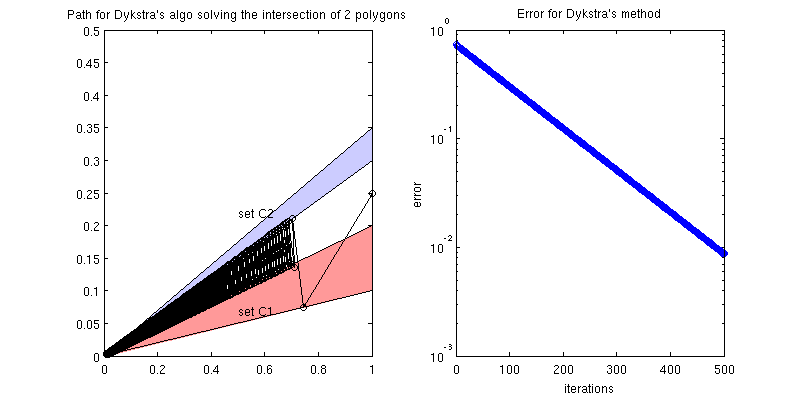With Dykstra’s algo (and alternating projections), we get very slow convergence, because of the very low angle between the two sets. Here is a zoom in on the graph of the iterates:

figure
fill( xx1, yy1,red);
hold on
fill( xx2, yy2,blue);
xlim( [0,mx] ); ylim( [0,.5*mx] );
text(.5,.07,'set C1');
text(.5,.22,'set C2');
plot( path(1,:), path(2,:),'ko-' )
title('Path for Dykstra''s algo solving the intersection of 2 polygons (zoom)');
xlim( [.25,.4] );
ylim( [.058,.1])## Plot all the errors together

figure
semilogy( out.err(:,1),'-' ,'linewidth',3);
hold all
semilogy( errHist_1,'-','linewidth',3);
semilogy( errHist,'--','linewidth',3);
legend('TFOCS','Alternating projection','Dykstra');
xlabel('iteration'); ylabel('error');## Demo with two circles that intersect at (0,0)

center1     = [0;.5];
center2     = -center1;

figure
axis equal
hold on
text(0,.5,'set C1');
text(0,-.5,'set C2');

offset1     = center1;
offset2     = center2;

x0          = [1; .2];

% and some simpler operators that we will use with alternating projections
% and with Dykstra
proj1   = @(x) radius1*(x-center1)/norm(x-center1) + center1;
proj2   = @(x) radius2*(x-center2)/norm(x-center2) + center2;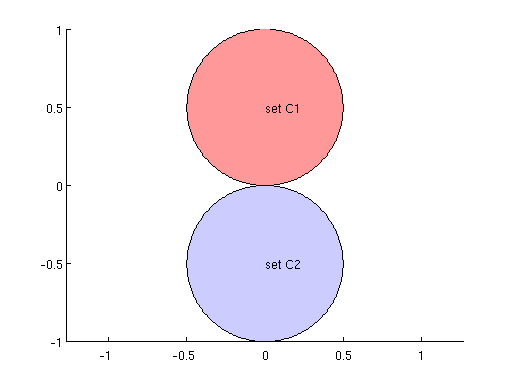## solve in TFOCS

opts.maxIts     = 300;
affineOperator  = {eye(N),offset1;eye(N),offset2};
dualProx        = {op1_d,op2_d};
% Solve in TFOCS:
[x,out,optsOut] = tfocs_SCD( [], affineOperator, dualProx , mu, x0, [], opts );

% Record the path:
path    = recordPoints();
path    = [x0,path];
% Plot:
figure
subplot(1,3,1);
hold on
text(0,.5,'set C1');
text(0,-.5,'set C2');
plot( path(1,:), path(2,:),'ko-','linewidth',2 )
title('Path for TFOCS solving the intersection of 2 circles');

subplot(1,3,2);
hold on
plot( path(1,:), path(2,:),'ko-','linewidth',2 )
xlim([0,.15]);
ylim([-.1,.1]);
title('(zoom)');

subplot(1,3,3);
semilogy( out.err,'o-')
title('Error of iterates for TFOCS method');
set(gcf, 'Position', [400 200 800 400]);
Auslender & Teboulle's single-projection method
Iter    Objective   |dx|/|x|    step      errors
----+----------------------------------+-------------------
20  | +4.54278e-07  5.76e-08  3.50e-07 | 1.03e-01 0.00e+00
40  | +4.77330e-07  7.02e-08  7.67e-07 | 6.54e-02 0.00e+00
60  | +4.87236e-07  6.88e-08  9.69e-07 | 5.00e-02 0.00e+00
80  | +4.92960e-07  7.36e-08  1.35e-06 | 4.12e-02 0.00e+00
100 | +4.96441e-07  4.53e-08  6.01e-07 | 3.58e-02 0.00e+00
120 | +4.99070e-07  5.29e-08  9.22e-07 | 3.17e-02 0.00e+00
140 | +5.01121e-07  3.21e-08  3.83e-07 | 2.86e-02 0.00e+00
160 | +5.02670e-07  4.33e-08  7.53e-07 | 2.62e-02 0.00e+00
180 | +5.03948e-07  3.34e-08  4.85e-07 | 2.43e-02 0.00e+00
200 | +5.05036e-07  4.42e-08  9.10e-07 | 2.26e-02 0.00e+00
220 | +5.05974e-07  2.64e-08  3.51e-07 | 2.12e-02 0.00e+00
240 | +5.06727e-07  3.52e-08  6.55e-07 | 2.01e-02 0.00e+00
260 | +5.07428e-07  2.81e-08  4.41e-07 | 1.90e-02 0.00e+00
280 | +5.08001e-07  3.32e-08  6.45e-07 | 1.81e-02 0.00e+00
300 | +5.08570e-07  4.60e-08  1.30e-06 | 1.73e-02 0.00e+00
Finished: Iteration limit reached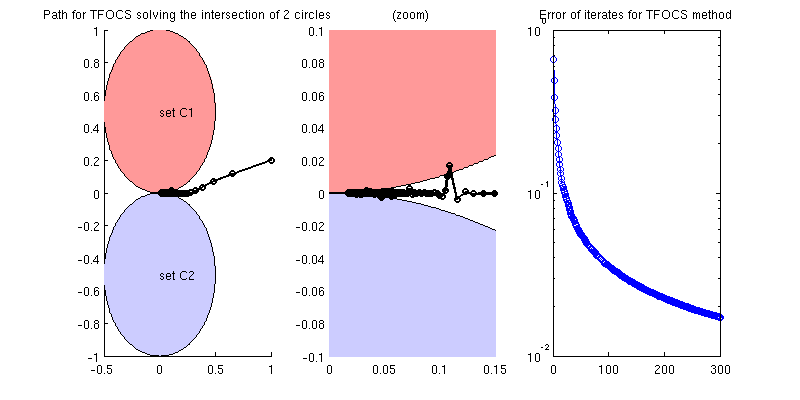## solve via alternating projection method

maxIts  = 300;
x       = x0;
path    = [x0];
errHist = [];
for k = 1:maxIts
% basic alternating projection method:
x   = proj1(x);
path= [path, x];
x   = proj2(x);
path= [path, x];
errHist     = [ errHist; err(x) ];
if ~mod(k,50)
fprintf('Iter %4d, error is %.2e\n', k, errHist(end) );
end
end
figure
subplot(1,3,1);
hold on
text(0,.5,'set C1');
text(0,-.5,'set C2');
plot( path(1,:), path(2,:),'ko-' )
title('Path for alternating projection method solving the intersection of 2 circles');

subplot(1,3,2);
hold on
plot( path(1,:), path(2,:),'ko-','linewidth',2 )
xlim([0,.15]);
ylim([-.1,.1]);
title('(zoom)');

subplot(1,3,3);
semilogy( errHist,'o-')
errHist_1 = errHist;
title('Error of iterates for alternating projection method');
set(gcf, 'Position', [400 200 800 400]);
Iter   50, error is 3.53e-02
Iter  100, error is 2.50e-02
Iter  150, error is 2.04e-02
Iter  200, error is 1.77e-02
Iter  250, error is 1.58e-02
Iter  300, error is 1.44e-02## solve via Dykstra’s algorithm

maxIts  = 300;
x       = x0;
[p,q]   = deal( 0*x0 );
path    = [x0];
errHist = [];
for k = 1:maxIts
% If x + p is feasible, the y = (x+p), so p=x+p-y = 0.
y   = proj1( x + p );
p   = x + p - y;
x   = proj2( y + q );
q   = y + q - x;
path = [path,y];
path = [path,x];
errHist     = [ errHist; err(x) ];
if ~mod(k,50)
fprintf('Iter %4d, error is %.2e\n', k, errHist(end) );
end
end
figure
subplot(1,3,1);
hold on
text(0,.5,'set C1');
text(0,-.5,'set C2');
plot( path(1,:), path(2,:),'ko-' )
title('Path for Dykstra''s algo solving the intersection of 2 circles');

subplot(1,3,2);
hold on
plot( path(1,:), path(2,:),'ko-','linewidth',2 )
xlim([0,.15]);
ylim([-.1,.1]);
title('(zoom)');

subplot(1,3,3);
semilogy( errHist,'o-')
title('Error of iterates for Dykstra''s algo');
set(gcf, 'Position', [400 200 800 400]);
Iter   50, error is 9.52e-02
Iter  100, error is 7.52e-02
Iter  150, error is 6.56e-02
Iter  200, error is 5.96e-02
Iter  250, error is 5.53e-02
Iter  300, error is 5.20e-02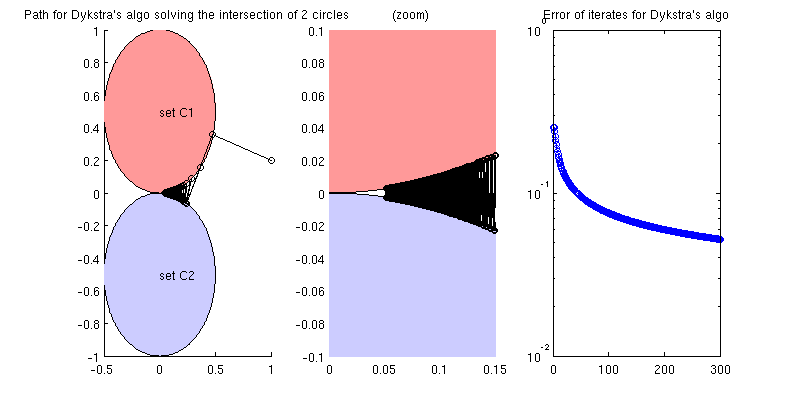## Plot all the errors together

figure
semilogy( out.err(:,1),'-' ,'linewidth',3);
hold all
semilogy( errHist_1,'-','linewidth',3);
semilogy( errHist,'--','linewidth',3);
legend('TFOCS','Alternating projection','Dykstra');
xlabel('iteration'); ylabel('error');Published with MATLAB® 7.12